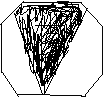SEARCH HOMEMath Central Quandaries & QueriesSuppose one vertex angle of a regular octagon is trisected as shown in the figure. Each side of the octagon measures 4 units. Find the area of the shaded area. Thanks for your time and effort i greatly appreciate it. chelsyChelsy,Chelsy's sketch

In the sketch I see, there isn't a trisected vertex angle. I assume that the written description is what you want?

Here is how to explore this problem. Draw an accurate regular octagon and figure out the interior angle at each vertex. (Hint: If the octagon is ABCDEFGH, extend AB and CD till they meet. What is the exterior angle?)

Now draw all the diagonals starting at one particular vertex. Identify any diagonals that are parallel with sides.

Now label all the angles in your diagram. Some strategies: spot right angles; spot isosceles triangles; if you know two angles of a triangle use the fact that the three angles add to 180 degrees; find pairs of angles that add up to one octagon interior angle.

If the edge BC is (as you said) 4 units, how long is the diagonal AD? (You may need Pythagoras' theorem and a couple new lines here.)

You should now know enough about octagons to answer your question easily (and a lot of others!) You can also guess something important about regular polygons and their diagonals in general.

Good Hunting!
RDMath Central is supported by the University of Regina and The Pacific Institute for the Mathematical Sciences.